# Plot_stat_map shows strange colormap behavior when plotting a thresholded image

I use `nistats` to perform a second-level analysis on sMRI data. I am using `nistats.thresholding.map_threshold` in order to obtain both an unthresholded statistical image and a corresponding threshold for this image. In my script I first used `nilearn.plotting.plot_stat_map` to plot my thresholded image (with `threshold == 'None'`):

``````plotting.plot_stat_map(stat_map_img=z_img_thresholded,
colorbar=True,
symmetric_cbar=True,
cmap='coolwarm',
threshold=None,
cut_coords=[1,11,8])
``````

However, this returns the following plot: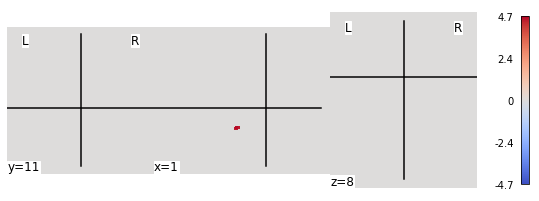However, when I used the unthresholded image and provided the corresponding threshold, the plot ‘looked fine’:

``````plotting.plot_stat_map(stat_map_img=z_img,
colorbar=True,
symmetric_cbar=True,
cmap='coolwarm',
threshold=threshold,
cut_coords=[1,11,8])
``````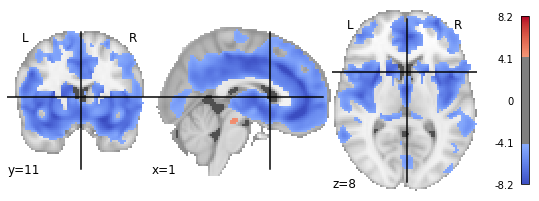This problem seems to have something to do with the used colorbar. Intuitively, I would expect both functions to plot the same plot, as plotting an already thresholded image vs. plotting an unthresholded image + providing the corresponding threshold is logically the same. Did I use the functions wrong, is this behavior wanted, or is this a bug?

can you try `threshold=1e-6` ?

``````plotting.plot_stat_map(stat_map_img=z_img_thresholded,
colorbar=True,
symmetric_cbar=True,
cmap='coolwarm',
threshold=1e-6,
cut_coords=[1,11,8])
``````

returns: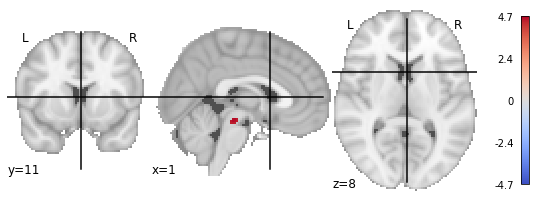With this, the background image ‘reappears’ but the colorbar scale is still different (-4.7 - 4.7 vs. -8.2 - 8.2)

thanks! can you also set `vmax=8.2`, or whatever other value

``````plotting.plot_stat_map(stat_map_img=z_img_thresholded,
colorbar=True,
symmetric_cbar=True,
vmax=8.2,
cmap='coolwarm',
threshold=1e-6,
cut_coords=[1,11,8])
``````

returns: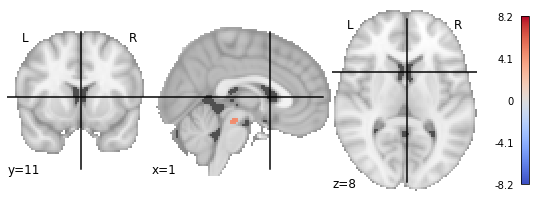thanks! it seems the thresholding kept only the positive part of the image

indeed looking at nistat’s code I don’t see negative values being handled
how did you call `map_threshold`?

actually this seems to have changed with nistats commit 6447ce1b39f3bd680daf1ccb0d3def6de653b177

if you pull nistats master and use the `two_sided` argument when calling `map_threshold` that should fix the problem

I call `map_threshold` with the following arguments.

ALPHA = 0.05
TWO_SIDED = False
HEIGHT_CONTROL = ‘fdr’
CLUSTER_THRESHOLD = 0

``````z_img_thresholded, threshold = map_threshold(stat_img=z_img,
alpha=ALPHA,
height_control=HEIGHT_CONTROL,
cluster_threshold=CLUSTER_THRESHOLD,
two_sided=TWO_SIDED)
``````

don’t you need `two_sided=True` ?

That ‘solved’ the problem. Calling `map_threshold` with `two_sided=True` and then calling

``````plotting.plot_stat_map(stat_map_img=z_img_thresholded,
colorbar=True,
symmetric_cbar=True,
vmax=8.2,
cmap='coolwarm',
threshold=1e-6,
cut_coords=[1,11,8])
``````

outputs: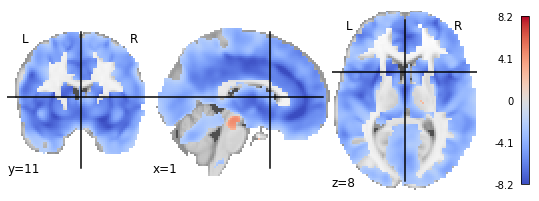However, this leaves me with the problem of my earlier post. @bthirion suggested to use `two_sided = False`, that’s why I used that setting for the analysis.

In general, in my analysis, I have specific hypotheses concerning the effect of groups, age and gender on grey matter density (older people have less grey matter densities, males have more than females, patients less than controls). Statistically, that would allow me to use a one-tailed-test, which means I am ‘allowed’ to divide my observed p-value by 2. I wanted follow this logic for my analyses.

When using `two_sided == True` it seems that the p-value is multiplied by the factor of 2, which follows a similar logic in the opposite direction (the test becomes more sensitive)

two_sided: Bool, optional,
Whether the thresholding should yield both positive and negative part of the maps. In that case, alpha is corrected by a factor of 2. Defaults to True.

I am not exactly sure, why` two_sided` controls the presence or absence of positive and negative values. Wouldn’t it make more sense to always present negative and positive values and let `two_sided ` only control the final value for my p-value?

I think you’re right, and this is the object of issues #444

If I’m not mistaken.

Sorry for introducing that bug. I need to work on the fix.